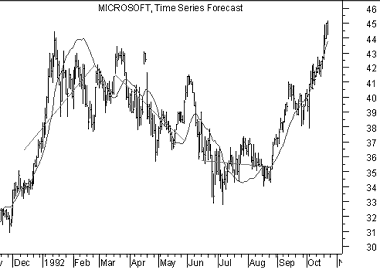# Technical Analysis from A to Z

by Steven B. Achelis

TIME SERIES FORCAST

Overview

The Time Series Forecast indicator displays the statistical trend of a security's price over a specified time period. The trend is based on linear regression analysis. Rather than plotting a straight linear regression trendline, the Time Series Forecast plots the last point of multiple linear regression trendlines. The resulting Time Series Forecast indicator is sometimes referred to as the "moving linear regression" indicator or the "regression oscillator."

Interpretation

The interpretation of a Time Series Forecast is identical to a moving average. However, the Time Series Forecast indicator has two advantages over classic moving averages.

Unlike a moving average, a Time Series Forecast does not exhibit as much delay when adjusting to price changes. Since the indicator is "fitting" itself to the data rather than averaging them, the Time Series Forecast is more responsive to price changes.

As the name suggests, you can use the Time Series Forecast to forecast the next period's price. This estimate is based on the trend of the security's prices over the period specified (e.g., 20 days). If the current trend continues, the value of the Time Series Forecast is a forecast of the next period's price.

Example

The following chart shows a 50-day Time Series Forecast of Microsoft's prices.I've also drawn three 50-day long linear regression trendlines. You can see that the ending point of each trendline is equal to the value of the Time Series Forecast.

Calculation

The Time Series Forecast is determined by calculating a linear regression trendline using the "least squares fit" method. The least squares fit technique fits a trendline to the data in the chart by minimizing the distance between the data points and the linear regression trendline. Click here to go to the formula for a linear regression trendline.

This online edition of Technical Analysis from A to Z is reproduced here with permission from the author and publisher.